# Difference between revisions of "10-327/Homework Assignment 7"

Read sections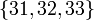$\{31,32,33\}$ in Munkres' textbook (Topology, 2nd edition). Remember that reading math isn't like reading a novel! If you read a novel and miss a few details most likely you'll still understand the novel. But if you miss a few details in a math text, often you'll miss everything that follows. So reading math takes reading and rereading and rerereading and a lot of thought about what you've read. Also, preread sections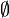$\emptyset$, just to get a feel for the future.

### Doing

Solve and submit the following problems from Munkres' book:

• Problem 1 on page 199.
• Problem 1 on page 205.
• Problems 1, 4, 5, 8, 9 on pages 212-213.

Remark. The following fact, which we will prove later, may be used without a proof: If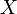$X$ is a topological space and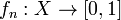$f_n:X\to[0,1]$ are continuous functions, then the sum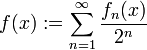$f(x):=\sum_{n=1}^\infty\frac{f_n(x)}{2^n}$ is convergent and defines a continuous function on$X$.

### Due date

This assignment is due at the end of class on Thursday, November 25, 2010.

 Dror's notes above / Student's notes below
• Question: In problem 1 p205, is asks us to show that any closed subspace of a normal space is also normal. Do we really need the condition that the subspace be closed? - Jdw
• Yes. Drorbn 19:14, 19 November 2010 (EST)

Questions by Kai Xwbdsb 21:26, 19 November 2010 (EST) were moved to Classnotes for Thursday November 18 as they are about that class and not about this assignment. Drorbn 06:03, 20 November 2010 (EST)

• Question. If we have a finite set of continuous function mapping from any topological space into the reals. Any linear combination of these continuous function is still continuous right? The proof is a little extension of 157 proof. This is used to prove the statement you mentioned above. -KaiXwbdsb 17:14, 20 November 2010 (EST)
• Any linear combination of functions from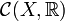$\mathcal{C}(X,\mathbb{R})$ (an uncountable set unless X is empty) is continuous. On its own, however, this proves nothing about infinite sums. Bcd 22:32, 21 November 2010 (EST)
• Question about 9. Is J any indexing set? Possibly uncountable? in the hint: A means any closed set? -Kai Xwbdsb 22:13, 20 November 2010 (EST)
• Yes,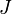$J$ is arbitrary and$A$ is closed. Drorbn 06:41, 22 November 2010 (EST)